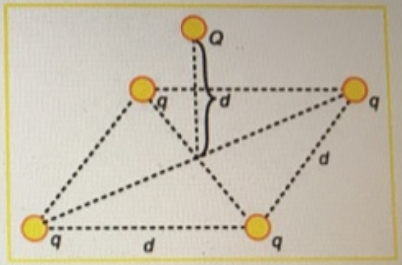# Problem: Four equal positive point charges q are fixed to the corners of a horizontal square. A fifth positive point charge Q is on a free particle of mass m positioned directly above the center of the square, at a height equal to the length d of one side of the square. Refer to the figure. Determine the magnitude of q in terms of defined quantities, the Coulomb constant, and g, if the system is in equilibrium.

###### FREE Expert Solution

Coulomb's law:

$\overline{){{\mathbf{F}}}_{{\mathbf{E}}}{\mathbf{=}}\frac{\mathbf{k}\mathbf{q}\mathbf{Q}}{{\mathbf{r}}^{\mathbf{2}}}}$

From the figure, each charge produces a vertical component. The vertical components are in the same axis as the weight. The weight is directed downward while the repulsive electric force is directed upward.

FE makes the hypotenuse. To solve for the opposite (vertical component of the electric force) we'll use the 'sin' trigonometric ratio. opp = hyp•sinθ

ΣF = 4FE•sinθ - mg = 0

96% (487 ratings)###### Problem Details

Four equal positive point charges q are fixed to the corners of a horizontal square. A fifth positive point charge Q is on a free particle of mass m positioned directly above the center of the square, at a height equal to the length d of one side of the square. Refer to the figure.Determine the magnitude of q in terms of defined quantities, the Coulomb constant, and g, if the system is in equilibrium.